# Efficiency

A certain device consumes 28 kW and delivers 14 kW. Find its efficiency.

p =  50 %

### Step-by-step explanation:

$p=\frac{14}{28}\cdot 100\mathrm{%}=50\mathrm{%}$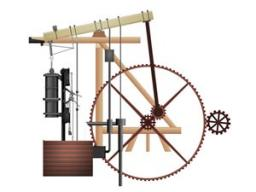Did you find an error or inaccuracy? Feel free to write us. Thank you!Tips to related online calculators
Check out our ratio calculator.

## Related math problems and questions:

• Efficiency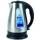What is the power output of a kettle 2 kW with an efficiency of 90%?
• Kilowatt-hours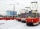If the Lewis family used 648 kilowatt-hours of electricity in 12 days, how many kilowatt-hours should they use in 24 days at the same usage rate?
• BonusThe gross wage was 1323 USD including 25% bonus. How many USD were bonuses?
• Power input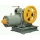The construction lift lifted a load weighing 300 kg to a height of 12 m with a uniform movement. How long has it taken for the load to be lifted if the efficiency is 75% and the engine has a power input of 5 kW?
• Motor physics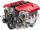A car is traveling at 98 km/h on the highway. The pulling power of the motor is 6 kN. Find the engine power in kW.
• VAT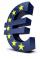John paid 1000 Euros in store for purchase. Calculate value added tax (VAT), which paid in purchase, if the VAT rate is 20%.
• Discount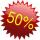Product has been discounted twice by 19%. What is the total discount given?
• RatioIncrease in the ratio 20:4 number 18.5.
• Bureaucracy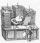23 people attended the office. 9 of them completed the questionnaire. What is the percentage of all people?
• TroopsThe route is long 147 km and the first-day first regiment went at an average speed of 12 km/h and journey back 21 km/h. The second day went second regiment the same route at an average speed of 22 km/h there and back. Which regiment will take route longer
• An orchard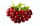In an orchard, there are 28 apple trees, 27 orange trees, and 14 cherry trees. What is the ratio of apple trees to cherry trees?
• Gain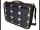Find the gain whenever the output power is 21x the input power.
• Farmer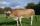The farmer farming 293 ha of arable land and the remaining 20% are meadows and pastures. How much land farmer owns?
• Percent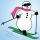From 99 children who participated in the ski course were 23 excellent skiers, 13 good and 20 average and the rest were beginners. Calculate this data in percentages.
• Ratio three numbers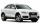Three numbers SUV are in the ratio 1:2:3. Their sum is 24. Find this numbers and write their add and sum.
• Percentages and numbers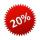How many percent is number 69 smaller than number 276?
• DonutsFind how many donuts each student will receive if you share 126 donuts in a ratio of 1:5:8